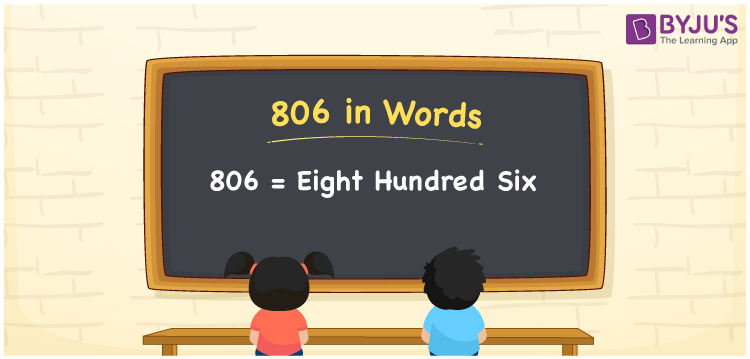# 806 in Words

We can write 806 in words as Eight hundred six. That means the number name or word form of 806 is Eight hundred six”. As we know, number names are used to represent or tell the given numbers to others. For example, you spent Rs. 806 for a feast in a diner, then you can say, “I spent Eight hundred six rupees for a feast in the diner”. Let’s learn how to derive the word form of 806 here in this article.

 806 in words Eight hundred six Eight hundred six in Numbers 806

## 806 in English Words

We generally write numbers in words with the help of the English alphabet. So, we spell 806 in English words as “Eight hundred six”.## How to Write 806 in Words?

The number 806 is a three-digit number so let’s make a place value chart in such a way that all the digits of 806 are defined with a specific place value.

 Hundreds Tens Ones 8 0 6

Here, ones = 6, tens = 0, hundreds = 6

These numbers can be expanded, as given below.

8 × Hundred + 0 × Ten + 6 × One

= 8 × 100 + 6 × 1

= 800 + 6

= Eight hundred + Six

= Eight hundred six

Therefore, 806 in words = Eight hundred six

As we know, 806 is a natural number that precedes 807 and succeeds 805.

806 in words – Eight hundred six

Is 806 an odd number? – No

Is 806 an even number? – Yes

Is 806 a prime number? – No

Is 806 a composite number? – Yes

Is 806 a perfect square number? – No

Is 806 a perfect cube number? – No

## Frequently Asked Questions on 806 in Words

Q1

### How do you write 806 in words?

We can write the number 806 in words as “Eight hundred six”.
Q2

### Write the value of 806 + 900 in words.

806 + 900 = 1706 Hence, we can write the value of 806 + 900, i.e., 1706 in words as One thousand seven hundred and six.
Q3

### How to write Rs. 806 in words on a cheque?

On a cheque, we generally write Rs. 806 in words as “Eight hundred six rupees only”.